# SQL Cast And Convert Statement

## Introduction

In this chapter, we will learn how to use a SQL Cast and convert statements with various options.

## Cast statement

The Cast statement is used to convert a data type variable or data from one data type to another data type. If the conversion fails, the function will return an error. Otherwise, it will return the converted value. The Cast statement provides a data type to a dynamic parameter (?) or a NULL value in SQL.

Syntax
1. CAST(expression AS datatype(length))

## Convert statement

The Convert statement is used to convert expressions from one type to another, in many cases there will be a need within a stored procedure or another routine to convert data from a datetime type to a varchar type. The Convert statement is used for such things. The Convert() function can be used to display date/time data in various formats in SQL datatype.

Syntax
1. CONVERT(data_type(length), expression, style)

## Using both Cast and Convert function

These examples retrieve the name of the OrderName for those OrderDetails that have a 1 as the first digit of OrderName, and converts their OrderId values to int.

Syntax
1. -- Use CAST
2.  USE sample;
3.  GO
4.  SELECT SUBSTRING(OrderName, 1, 40) AS OrderName, orderAddress
5.  FROM OrderDetails
6.  WHERE CAST( OrderId AS intLIKE  '10%';
7.  GO
8.
9.  -- Use CONVERT.
10.  USE sample;
11.  GO
12.  SELECT SUBSTRING(OrderName, 1, 40) AS OrderName , orderAddress
13.  FROM OrderDetails
14.  WHERE CONVERT(int, OrderId) LIKE '8%';
15.  GO
Example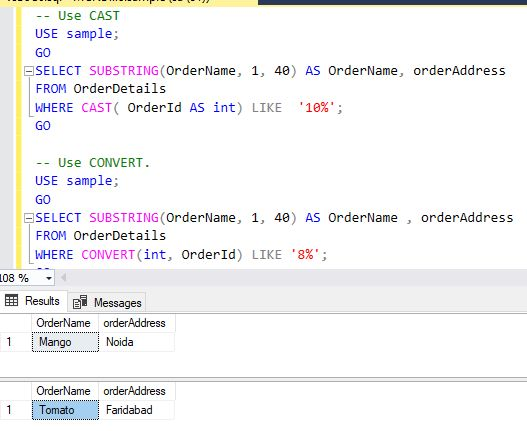## The Cast statement with arithmetic operators

In this example, we can calculate a single column computation (Computed) by dividing the total OrderId by the OrderDetails. This value is rounded to the nearest whole number and is then Cast to a data type.

Syntax
1. USE sample ;
2. GO
3. SELECT CAST(ROUND(OrderId, 0) AS intAS Computed
4. FROM OrderDetails
5. WHERE OrderId != 0;
6. GO
Example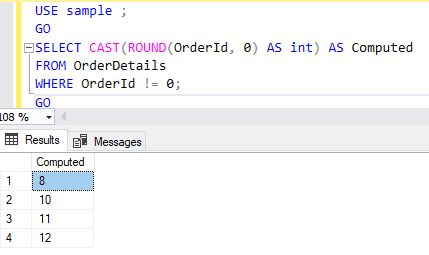## Using the Cast to concatenate statement

This example concatenates noncharacter expressions by using Cast. It uses the Sample database.

Syntax
1. SELECT 'The Order Details ' + CAST(OrderName AS varchar(12)) AS OrderCity
2. FROM OrderDetails
3. WHERE OrderId  BETWEEN 8 AND 10;
Example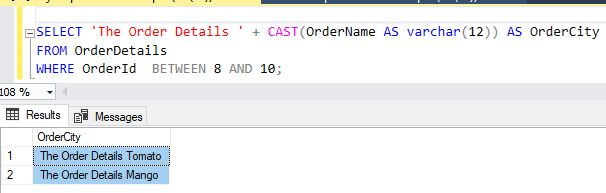## Using Cast to produce more readable text statement

This example uses Cast in the Select list, to convert the OrderName column to a char(50) column.

Syntax
1. SELECT DISTINCT CAST(OrderName AS char(50)) AS Name
2. FROM OrderDetails
3. WHERE OrderName LIKE 'Mango';
Example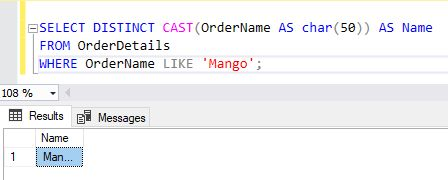## Using Cast with the Like clause statement

This example converts the EmpName, EmpAddress, EmpCity column OrderIdvalues to data type Int, and then to data type char(200)so that the  Like clause can use it.

Syntax
1. USE sample;
2. GO
4. FROM EmployeeDetail AS p
5. JOIN OrderDetails AS s
6.     ON s.OrderId = s.OrderId
7. WHERE CAST(CAST(s.OrderId AS intAS char(200)) LIKE '1%';
8. GO
Example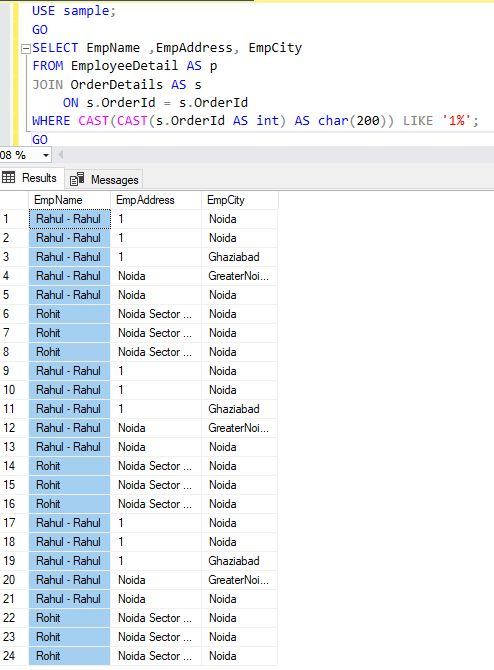## Using Convert or Cast with typed XML statement

These examples show the use of Convert to convert data to typed XML by using the XML data type and columns SQL Server.

This statement converts a string with white space text and markup into typed XML and removes all insignificant white space (boundary white space between nodes).

Syntax
1. SELECT CONVERT(XML, '<root><child/></root>')
Example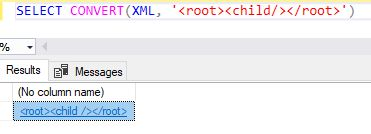This example converts a similar string with white space, text, and markup into typed XML and preserves insignificant white space boundary white space between nodes in the Convert statement.

Syntax
1. SELECT CONVERT(XML, '<root>          <child/>         </root>', 1)
This example casts a string with white space, text, and markup into typed XML

Example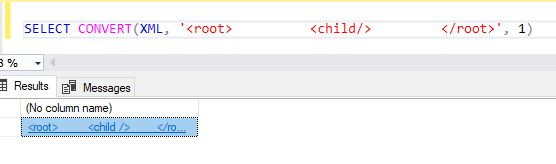This example casts a string with white space, text, and markup into typed XML in SQL.

Example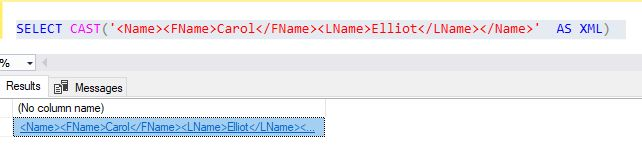## Using Cast and Convert with the DateTime data statement

The cast and convert Starting with Getdate() values, this example displays the current date and time, uses Cast to change the current date and time to a character dataType, and then uses Convert to display the date and time in the ISO 8601 format in SQL

Syntax
1. SELECT
2.    GETDATE() AS UnconvertedDateTime,
3.    CAST(GETDATE() AS nvarchar(40)) AS UsingCast,
4.    CONVERT(nvarchar(40), GETDATE(), 126) AS UsingConvertTo_ISO8601  ;
5. GO
Example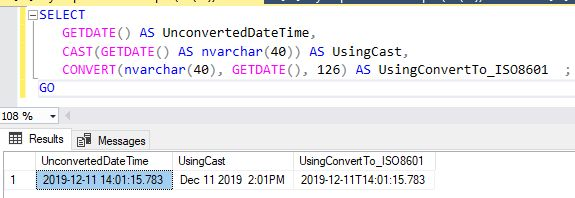This statement  is approximately the opposite of the previous statement

This example displays a date and time as character data, uses Cast to change the character data to the datetime data type, and then uses  Convert to change the character data to the datetime data type.

Syntax
1. SELECT
2.    '2006-04-25T15:50:59.997' AS UnconvertedText,
3.    CAST('2006-04-25T15:50:59.997' AS datetime) AS UsingCast,
4.    CONVERT(datetime, '2006-04-25T15:50:59.997', 126) AS UsingConvertFrom_ISO8601 ;
5. GO
Example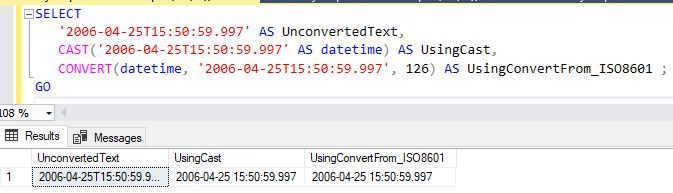## Using Convert  with binary and character data statement

This example shows the results of binary and character data conversion, using different styles.

Syntax
1. SELECT CONVERT(char(8), 0x4E616d65, 0) AS [Style 0, binary to character];
Example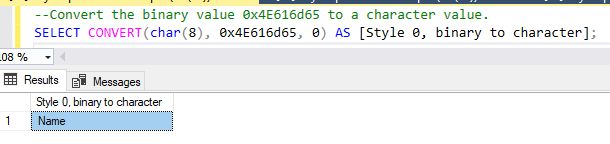This example shows that style 1 can force a truncation result.  The characters 0x in the result set force the truncation.

Syntax
1. SELECT CONVERT(char(8), 0x4E616d65, 1) AS [Style 1 binary to character]
Example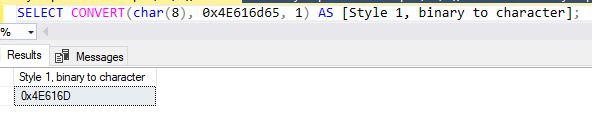## Converting date and time data types

This example shows the conversion of date, time, and datetime data types. ]]

Syntax
1. DECLARE @d1 date, @t1 time, @dt1 datetime;
2. SET @d1 = GETDATE();
3. SET @t1 = GETDATE();
4. SET @dt1 = GETDATE();
5. SET @d1 = GETDATE();
6. -- When converting date to datetime the minutes portion becomes zero.
7. SELECT @d1 AS [date], CAST (@d1 AS datetime) AS [date as datetime];
8. -- When converting time to datetime the date portion becomes zero
9. -- which converts to January 1, 1900.
10. SELECT @t1 AS [time], CAST (@t1 AS datetime) AS [time as datetime];
11. -- When converting datetime to date or time non-applicable portion is dropped.
12. SELECT @dt1 AS [datetime], CAST (@dt1 AS dateAS [datetime as date],
13.    CAST (@dt1 AS timeAS [datetime as time];

## Using convert with datetime data in different formats

Starting with getdate() values, this example uses Convert to display of all the date and time styles in section Date and Time styles of this article.

Effects of data type precedence in the allowed conversions statement.

The following example defines a variable of type Varchar, assigns an integer value to the variable, then selects a concatenation of the variable with a string.

Syntax
1. DECLARE @string varchar(10);
2. SET @string = 1;
3. SELECT @string + ' is a string.' AS Result
Example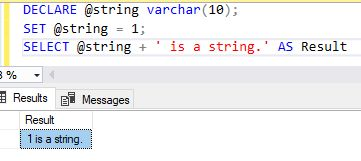The int value of 1 was converted to varchar.

This example shows a similar query using an int variable instead.

Syntax
1. DECLARE @notastring int;
2. SET @notastring = '1';
3. SELECT @notastring + ' is not a string.' AS Result
In this case, the Select statement will throw the following error.

ExampleThis statement In order to evaluate the expression @notastring + is not a string SQL Server statement and needs to follow the rules of data type precedence to complete the implicit conversion before the result of the expression can be calculated.

Because int has higher precedence than varchar SQL Server attempts to convert the string to an integer and fails because this string cannot be converted to an integer.

If we provide a string that can be converted this statement will succeed, as seen in the following example.

Syntax
1. DECLARE @notastring int;
2. SET @notastring = '1';
3. SELECT @notastring + '1
In this case, the string '1' can be converted to the integer value 1 so this Select statement will return the value 2. When the data types provided are integers, the + operator becomes an additional mathematical operator, rather than a string concatenation in SQL statement.

## Summary

In this chapter, we learned how to use SQL Stored Procedures with various options.
Next » Create Stored Procedure In MySQL